AN137 - Using the MagAlpha Serial Interface Advanced FeaturesGet valuable resources straight to your inbox - sent out once per month

INTRODUCTION

This MagAlpha serial interface has some advanced features that are not listed in the datasheets. This application note explains what they are and how to use them.

PARITY BIT (SPI AND SSI)

This parity bit, or check bit, is a bit added to a string of binary code to ensure that the total number of 1 in the string is even (see Table 1). Parity bits are used as error detecting code. The MagAlpha transmits the parity bit at the 17th clock edge.

 16-Bits Angle Value Number of Bits Set to "1" 16-Bits Angle Value + 1-Bit Parity Check 0000000000000000 0 00000000000000000 1000110001100010 6 10001100011000100 1000110001100011 7 10001100011000111 0101110100000000 5 01011101000000001

Table 1: Parity Bit Behavior Overview 16-Bits Angle Value

Figure 1 through Figure 4 show SPI and SSI frames with and without parity bits.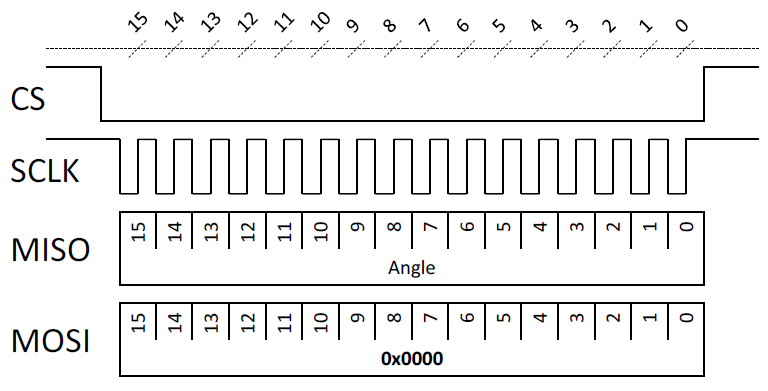Figure 1: SPI Frame without Parity Bit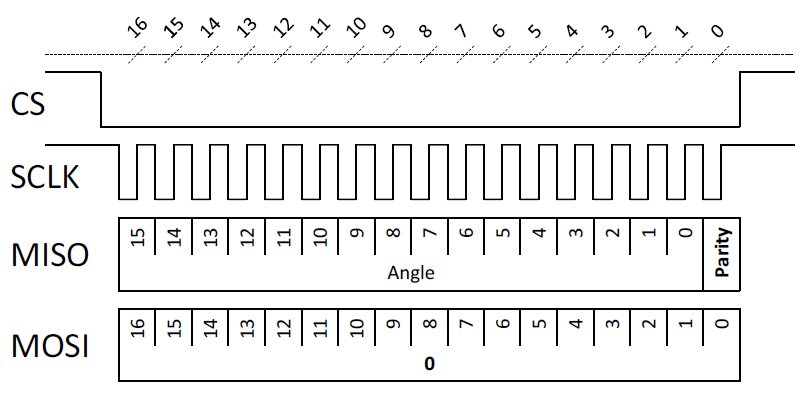Figure 2: SPI Frame with Parity Bit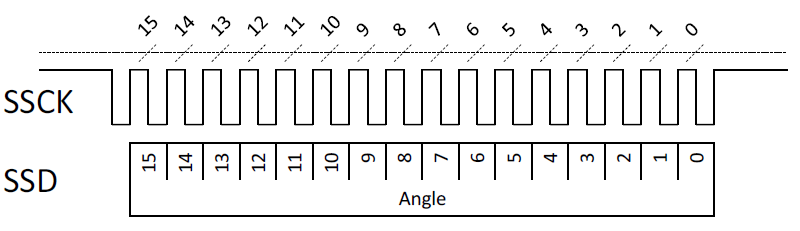Figure 3: SSI Frame without Parity Bit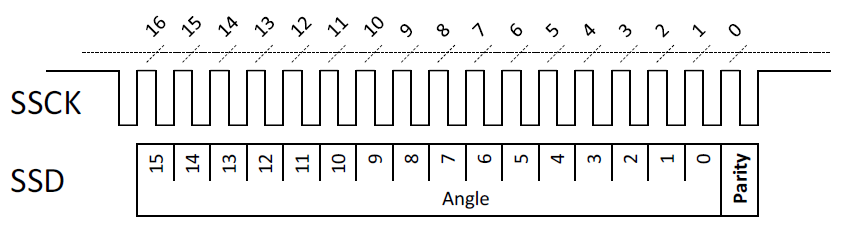Figure 4: SSI Frame with Parity Bit

It is possible to concatenate multiple read or write register commands together to save time. To concatenate read/write frames together, simply send the next read/write command while reading the previous register value.

The last SPI frame of the sequence must send 0x0000 to the MagAlpha to set the sensor in angle readback mode (see Figure 5). If this is not done, the next read angle operation will return a register value instead of an angle.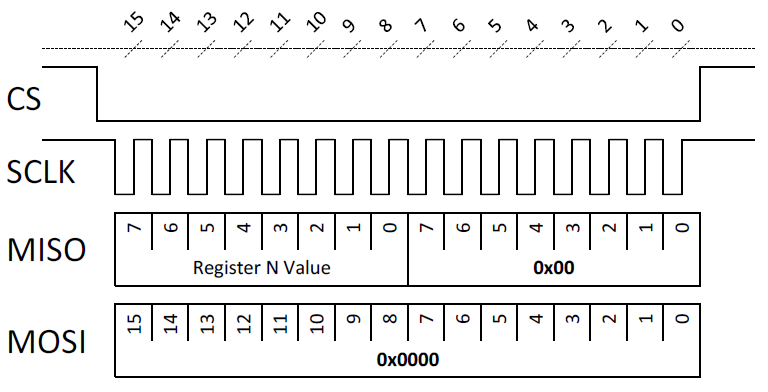Figure 5: SPI Last Concatenated Frame of the Sequence Must Send 0x0000 Value to the Sensor

Figure 6 to Figure 9 show the difference between the standard read/write commands and the concatenated commands for both SPI and SSI protocols.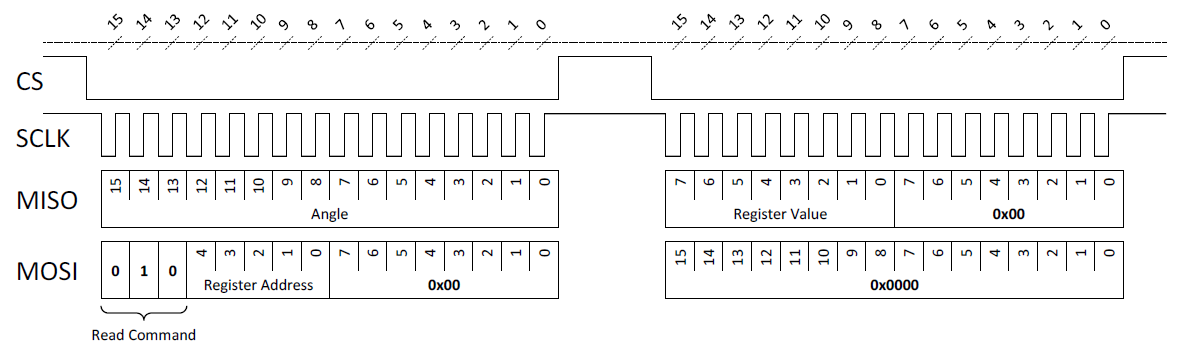Figure 6: SPI Standard Read Command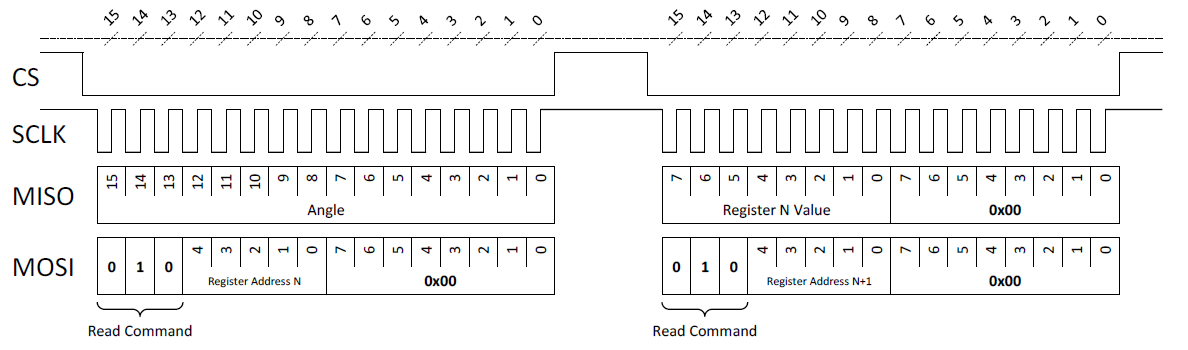Figure 7: SPI Concatenated Read Commands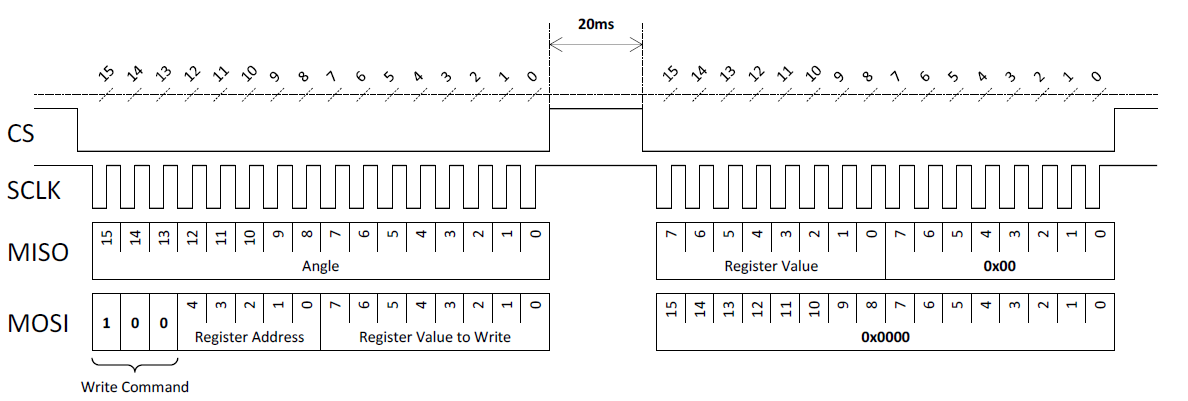Figure 8: SPI Standard Write Command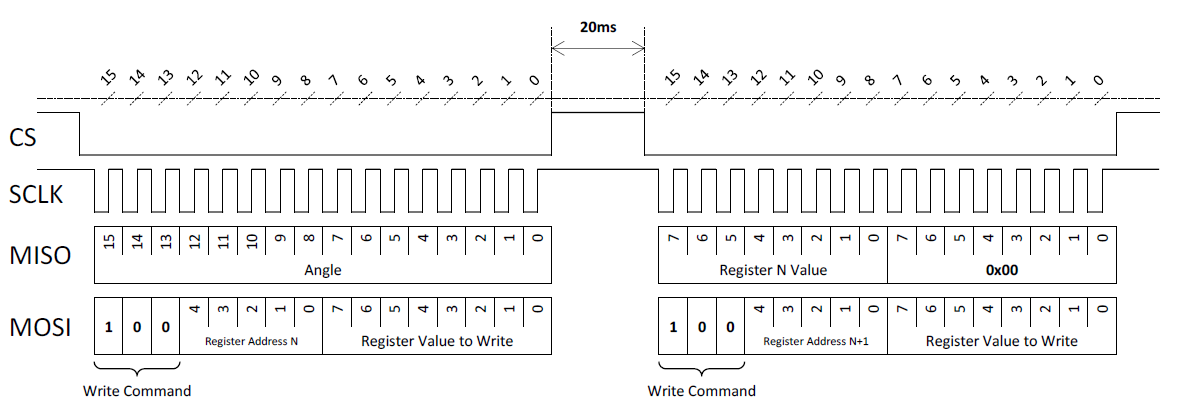Figure 9: SPI Concatenated Write Commands

READ ANGLE WITH SSI USING SPI HOST

It is possible to emulate an SSI host by using an SPI interface in Mode 2 (CPOL 1, CPHA 0) (see Table 2 and Table 3).

Please note that not all SPI Mode 2 will work out of the box. Some MCUs propagate the MSB bit with the CS falling edge instead of with the first clock rising edge and therefore do not add an extra clock to the SPI frame. In this case, it is required to add an extra clock count manually to read the correct number of bits (17 clock count to read a 16-bit angle) (see Figure 10).

If adding an extra bit at the beginning of the frame, do not forget to mask out the MSB bit (see the pseudo code below).

angle = spi17bitsOutputValue & 0x0FF;

 SPI SSI Clock SCLK SSCK Data from the sensor to the host MISO SSD

Table 2: SSI SPI Signal Mapping

 SPI Mode 2 SSI Clock idle state High High Data propagation Rising edge Rising edge Data capture edge Falling edge Falling edge

Table 3: SSI Emulation with SPI Mode 2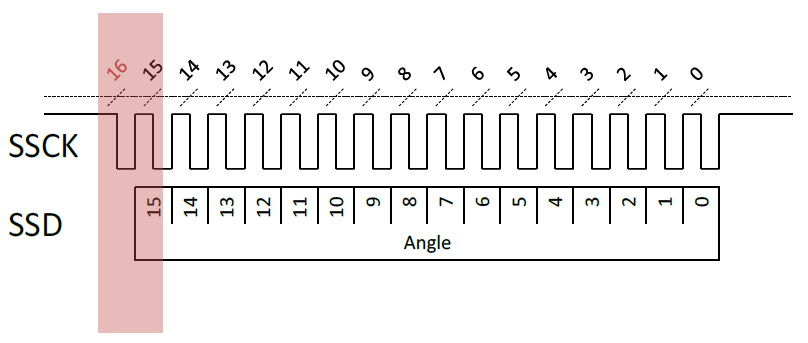Figure 10: Possible Clock Count Issue with SSI Emulation